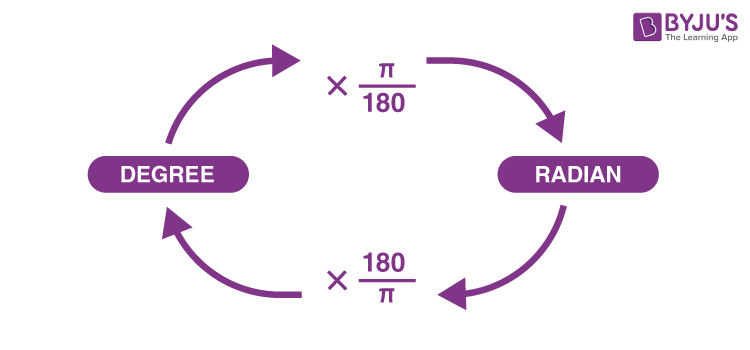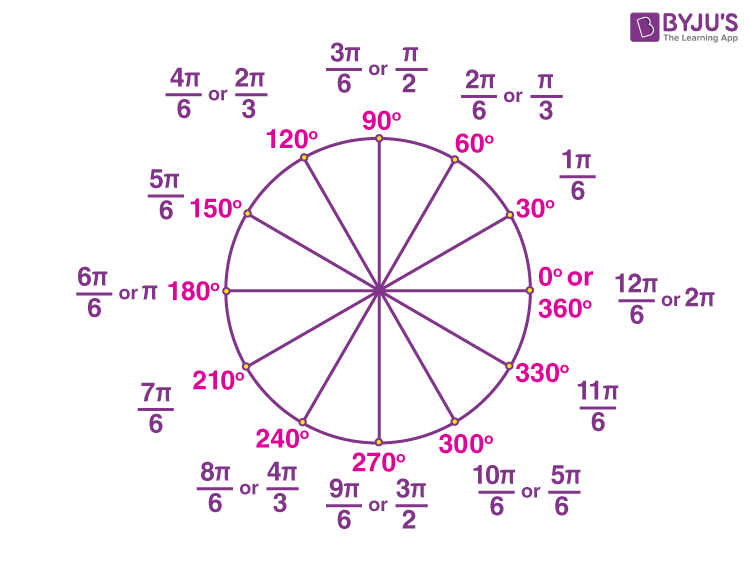# Relation Between Degree And Radian

The amount of revolution made to get the terminal side from the initial side is called the measure of an angle. There are different units for measuring angles. The most commonly used units of measurement of an angle are degree measure and radian measure. The relation between degree and radian measures of angles is used to convert the given measurement of an angle from one unit to another unit. Before understanding the relation between degree measure and radian measure, let us recall the degree and radian measure.

Degree measure

If a rotation from the initial side to terminal side is (1/360)th of a revolution, then the angle is said to have a measure of one degree, written as 1°.

Angle subtended at the centre by an arc of length 1 unit in a unit circle is said to have a measure of 1 radian.

## What is The Relation between Degree and Radian?

A complete angle of a circle is 360 degrees of 2π radians. This is the basis for converting the measures of angles from one unit to another. That means a circle subtends an angle whose radian measure is 2π and its degree measure is 360° at the centre. This can be written as:

or

The above relationship allows us to formulate a radian measure in degree measure and a degree measure in radian measure. We know that the approximate value of pi (π) is 22/7. By substituting this value in the above relation, we get;

1 radian = 180°/π = 57° 16′ (approx)

Also,The relation between degree measures and radian measures of some standard angles can be observed from the below figure:The most commonly used conversions from degrees to radians are tabulated below:

### Relation Between Radian Degree and Minutes

The relation among radians, degrees and minutes can be defined from the following:

1 radian = 180°/π = 57° 16′ (approx)

Here,

1° = 60′ (1 degree = 60 minutes)

1′ = 60″ (1 minute = 60 seconds)

That means, one-sixtieth of a degree is called a minute and one-sixtieth of a minute is called a second.

Using this relationship, we can convert any measure of angle to other units of measuring angles.

### Solved Examples

Example 1: Convert 135 degrees to radians.

Solution:

135° = 135° × (π/180°)

Or

Example 2: Convert 6 radians into the degree measure.

Solution:

We know that,

6 radian = 6 × (180°/π)

= (6 × 180°)/(22/7)

= (1080° × 7)/22

= 343 7/11 degrees

This can be further calculated as:

343 7/11 degrees = 343° + [(7 × 60)/11] minute

= 343° + 38′ + (2/11) minute

= 343° + 38′ + 10.9″

= 343° 38′ 11″ (approx)

Therefore, 6 radians = 343° 38′ 11″ (approx).

For more information on different units of measurement of angles and the relationship between them, visit www.byjus.com today!